### POPULATION SPEARMAN’s and KENDALL’S RANK CORRELATION COEFFICIENTS:

A population version of Spearman’s rank correlation has been defined in the case of continuous variables.

Consider a population distributed according to two variates $$X_1$$ and $$X_2.$$ Two members $$(X_1, X_2)$$ and $$(X_1', X_2')$$ of the population will be called concordant if:

$X_1 < X_1', \; X_2 < X_2' \text{ OR } X_1 > X_1',\; X_2 > X_2'.$

They will be called discordant if:

$X_1 < X_1', \; X_2 > X_2' \text{ OR } X_1 > X_1',\; X_2 < X_2'.$ The probabilities of concordance and discordance are denoted with $$P_c,$$ and $$P_d$$ respectively. The population version of Spearman’s $$\rho$$ is defined as proportional to the difference between the probability of concordance, and the probability of discordance for two vectors $$(X_1, X_2)$$ and $$(X_1', X_2'),$$ where $$(X_1, X_2)$$ has distribution $$F_{X_1X_2}$$ with marginal distribution functions $$F_{X_1}$$ and $$F_{X_2}$$ and $$X_1$$, $$X_2$$ are independent, and $$(X_1, X_2)$$ and $$(X_1', X_2')$$ are independent.

$\rho = 3 \left(\Pr[(X_1 − X_1')(X_2 − X_2') > 0] − \Pr[(X_1 − X_1')(X_2 − X_2') < 0]\right)$ The above definition is valid only for populations for which the probabilities of $$X_1 = X_1'$$ or $$X_2 = X_2'$$ are zero. The main types of such populations are an infinite population with both $$X_1$$ and $$X_2$$ distributed continuously, or a finite population where $$X_1$$ and $$X_2$$ have disjoint ranges.

This seems virtually identical to the concept of population Kendall’s tau:

In trying to illustrate pictorially the intuition behind the definition of the population Kendall’s tau as

$\tau (X_1, X_2) =\Pr\left[(X_1-X_1')\,(X_2 - X_2') >0 \right]- \Pr\left[(X_1-X_1')\,(X_2 - X_2') <0 \right]\tag 1$

and the relationship both 1. Between the iid random vector $$(X_1,X_2)$$ and its copy $$(X_1', X_2')$$; as well as 2. Between these random vectors (2-tuples of dependent random variables) and their joint and marginal densities and distributions (pdf and cdf’s), we can take a look at a somewhat illustrative value of $$X_1$$ in a bivariate normal distribution with a covariance $$\mathrm{cov}(X_1,X_2)=0.2.$$

In the example, $$X_1\sim N(0,0.25)$$ and $$X_2 \sim N(0,0.25).$$ The Pearson correlation is, therefore,

$\rho(X_1,X_2)=\frac{\mathrm{cov}(X_1,X_2)}{\sigma_{X_1}\,\sigma_{X_2}}=\frac{0.2}{\sqrt{0.25\times0.25}}=0.8.$

If we consider an illustrative draw from $$X_1$$ towards the left of the distribution, e.g. $$x_1=-1,$$ the conditional mean of $$X_2$$ given this value of $$X_1$$ will be given by

\begin{align} \mathbb E(X_2|X_1=x)=\mu_Y+\rho \dfrac{\sigma_Y}{\sigma_X}(x-\mu_X)= -0.8 \end{align}

and these conditional mean values will increase from left to right linearly as on the following plot: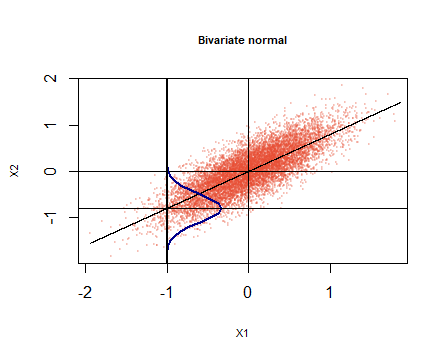and assuming constant variance, its value will be $$\mathrm{var}(X_2\vert X_1=x)=\sigma^2_{X_2}\,(1-\rho^2)=0.09$$

Now, looking at the first part of equation (1), $$(X_1-X_1')$$ will be negative whenever the independent draw from the identical rv $$X_1'$$ is less negative than $$x_1,$$ which is highly probable: $$\Pr(x_2 > -1) = 0.977.$$

Looking at second term of the multiplication, i.e. $$(X_2-X_2'),$$ we know that $$\mathbb E[X_2\vert X_1=-1]$$ is below the mean of the marginal distribution of $$X_2,$$ which was designed to be $$\mu_{X_2}=0.$$

Therefore, precisely because it is highly likely that $$x_1' > x_1,$$ rendering $$(X_1-X_1')<0,$$ it is also going to be more likely for $$x_2'>x_2,$$ since it will more probably come from a conditional normal distribution with $$\mathbb E[X_2'\vert X_1'=x_1' ] > \mathbb E[X_2\vert X_1=-1 ],$$ in which case $(X_2-X_2’)$ will also be negative, rendering $$(X_1-X_1')\,(X_2 - X_2') >0.$$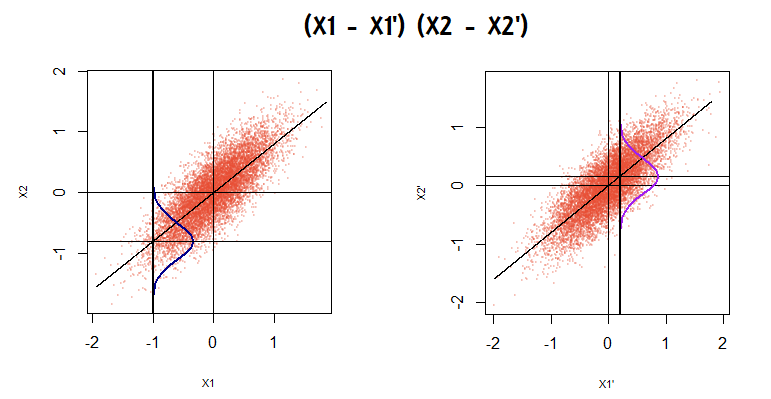The same argument (inverted) holds if we were to look at a value of $$x_1 =+1.$$

Therefore, the positive correlation imposed on this bivariate normal, would indeed result in a positive Kendall $$\tau$$ if we swept (integrate) from $$-\infty$$ to $$+\infty,$$ to the extent that $$\Pr\left[(X_1-X_1')\,(X_2 - X_2') >0 \right]> \Pr\left[(X_1-X_1')\,(X_2 - X_2') <0 \right].$$

The inverse would be easy to show if the correlation decided upon had been negative.

### COPULAS:

The objective is to look at dependency structure in a vector of random variables, knowing their marginal distributions.

We want to convert the random variables and convert them to $$U(0,1).$$

It is well known that a real-valued, continuous, and strictly monotone function of a single variable possesses an inverse on its range. It is also known that one can drop the assumptions of continuity and strict monotonicity (even the assumption of considering points in the range) to obtain the notion of a generalized inverse. One can often work with generalized inverses as one does with ordinary inverses.

For an increasing function $$T : \mathbb R \to \mathbb R$$ with $$T(−\infty) = \lim_{x \downarrow −\infty} T(x)$$ and $$T(\infty) =\lim_{x \uparrow \infty} T(x),$$ the generalized inverse

$$T^- : \mathbb R \to \bar{\mathbb R} = [−\infty, \infty]$$

of $$T$$ is defined by

$T^-(y)=\inf\{x\in \mathbb R: T(x) \geq y\}, y\in \mathbb R,$

with the convention that $$\inf \emptyset =\infty.$$ If $$T:\mathbb R\to [0,1]$$ is a distribution function, $$T^-:[0,1]\to \bar {\mathbb R}$$ is also called a quantile function of $$T.$$

In this context $$T$$ is the cdf $$F.$$ We have that $$F(F^-(y))=y.$$ And $$X \sim F^-(u).$$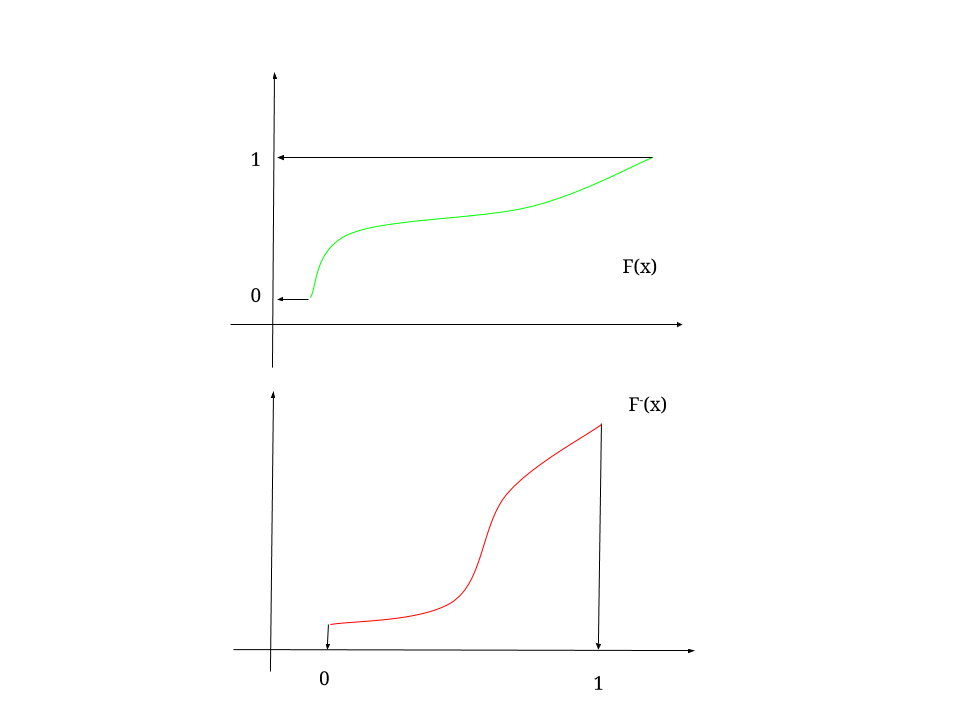[From here on, instead of $$y$$ we will use $$u$$]

So if we have a vector of random variables, $$\{X_1, \dots,X_n\}$$ and we think of them as the variable $$u,$$ we have that $$X_i = F^-_i(u)$$ and $$F(X_i) = U(0,1).$$ So each variable will be transformed as $$\{F_1,\dots,F_n\}.$$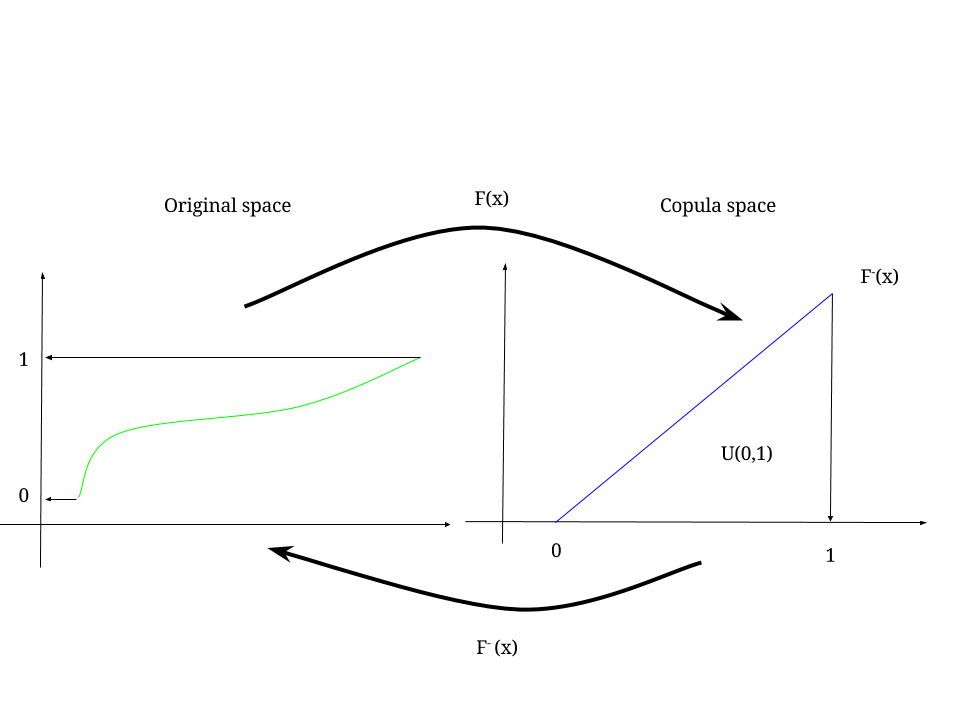We can say that $$F_i(X_i) \sim U_i$$ and $$F_i^- (U_i) \sim X_i.$$

We can look at the vector $$\vec u =(F_1(X_1), \dots, F_n(X_n)),$$ and call the joint distribution of vector $$\vec u$$ the copula:

$\Pr(U_1 \leq u_1, \dots, U_n \leq u_n).$

So the copula, $$\color{red}C$$ is a joint distribution function on $$[0,1]^d$$ with marginal distributions standard uniform: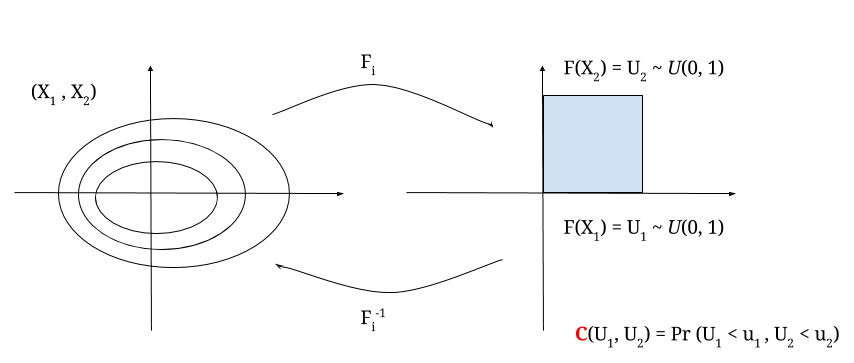For example, if we assume independence between $$X_i$$ variables,

$C(\vec u) = \Pr(U_1 \leq u_1, \dots, U_d \leq u_d) = \Pr(F_1(X_1)\leq u_1, \dots, F_d(X_d) \leq u_d)=\Pi_{i=1}^d (F_i(X_i)\leq u_i)= \Pi_{i=1}^d u_i.$

This is the independence copula.

In the case of a bivariate independent copula (here), $$C(u,v)=uv,$$ the plot will be